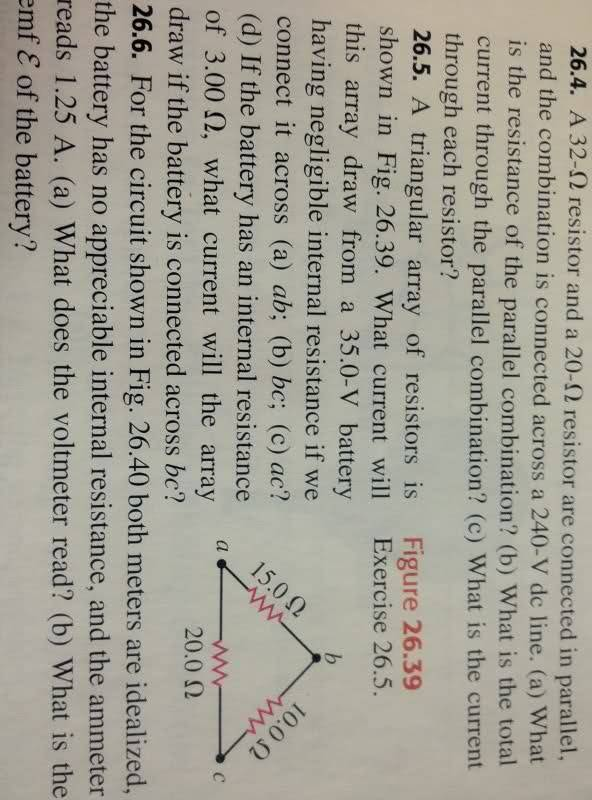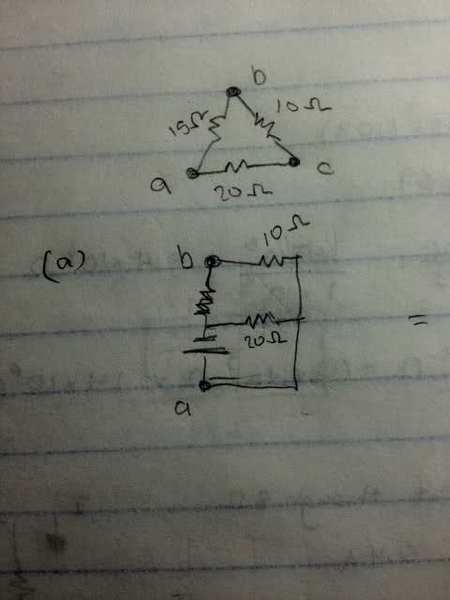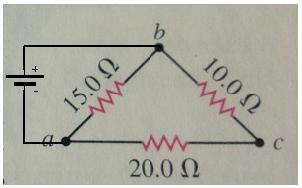# Triangle Circuit

I am not sure how the configuration would look like if we add a voltage across these points. I have drawn out what I think it would look like after a potential is applied across Vab. My question is, is this drawing correct?? What about Vbc and Vac?

This is question 26.5.And here is my attempt for (a)Once I figure out the configuration, I can easily add up the R_eq and find the I from that. But I need to make sure my configuration is correct!

For a diagram for part (a), the voltage source should be drawn between nodes a and b. You've not labeled node a correctly in the second diagram.

What should the 20ohm be connected to?

If the instructions say to put the voltage source between nodes a and b, then that's where you connect it!Ohhhhh, duh... Thanks!